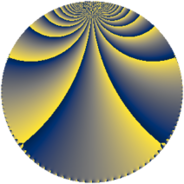# Properties

 Label 1344.4.bdLevel $1344$ Weight $4$ Character orbit 1344.bd Rep. character $\chi_{1344}(95,\cdot)$ Character field $\Q(\zeta_{6})$ Dimension $384$ Sturm bound $1024$

# Related objects

## Defining parameters

 Level: $$N$$ $$=$$ $$1344 = 2^{6} \cdot 3 \cdot 7$$ Weight: $$k$$ $$=$$ $$4$$ Character orbit: $$[\chi]$$ $$=$$ 1344.bd (of order $$6$$ and degree $$2$$) Character conductor: $$\operatorname{cond}(\chi)$$ $$=$$ $$168$$ Character field: $$\Q(\zeta_{6})$$ Sturm bound: $$1024$$

## Dimensions

The following table gives the dimensions of various subspaces of $$M_{4}(1344, [\chi])$$.

Total New Old
Modular forms 1584 384 1200
Cusp forms 1488 384 1104
Eisenstein series 96 0 96

## Trace form

 $$384q + O(q^{10})$$ $$384q - 4800q^{25} - 720q^{49} - 432q^{73} - 3696q^{81} + 15264q^{97} + O(q^{100})$$

## Decomposition of $$S_{4}^{\mathrm{new}}(1344, [\chi])$$ into newform subspaces

The newforms in this space have not yet been added to the LMFDB.

## Decomposition of $$S_{4}^{\mathrm{old}}(1344, [\chi])$$ into lower level spaces

$$S_{4}^{\mathrm{old}}(1344, [\chi]) \cong$$ $$S_{4}^{\mathrm{new}}(168, [\chi])$$$$^{\oplus 4}$$$$\oplus$$$$S_{4}^{\mathrm{new}}(672, [\chi])$$$$^{\oplus 2}$$